# Frank Solutions for Class 10 Maths Chapter 25 Probability

Frank Solutions for Class 10 Maths Chapter 25 Probability are provided here. While solving the exercise questions from Frank textbooks, students often face difficulty and eventually pile up their doubts. Our expert faculty formulated these solutions to assist students in their exam preparation to attain good marks in the board exam. Practise Frank Solutions for Class 10 Maths Chapter 25 regularly to score good marks in Maths.

In Chapter 25 – Probability, solutions are provided in a step-wise manner, which makes it easier to understand the difficult problems. In our daily life, we use probability to make decisions when we don’t know exactly what the output would be. Most of the time, we won’t perform actual probability, but instead, we use subjective probability to make judgement calls and determine the best course of action.

## Download the PDF of Frank Solutions for Class 10 Maths Chapter 25 Probability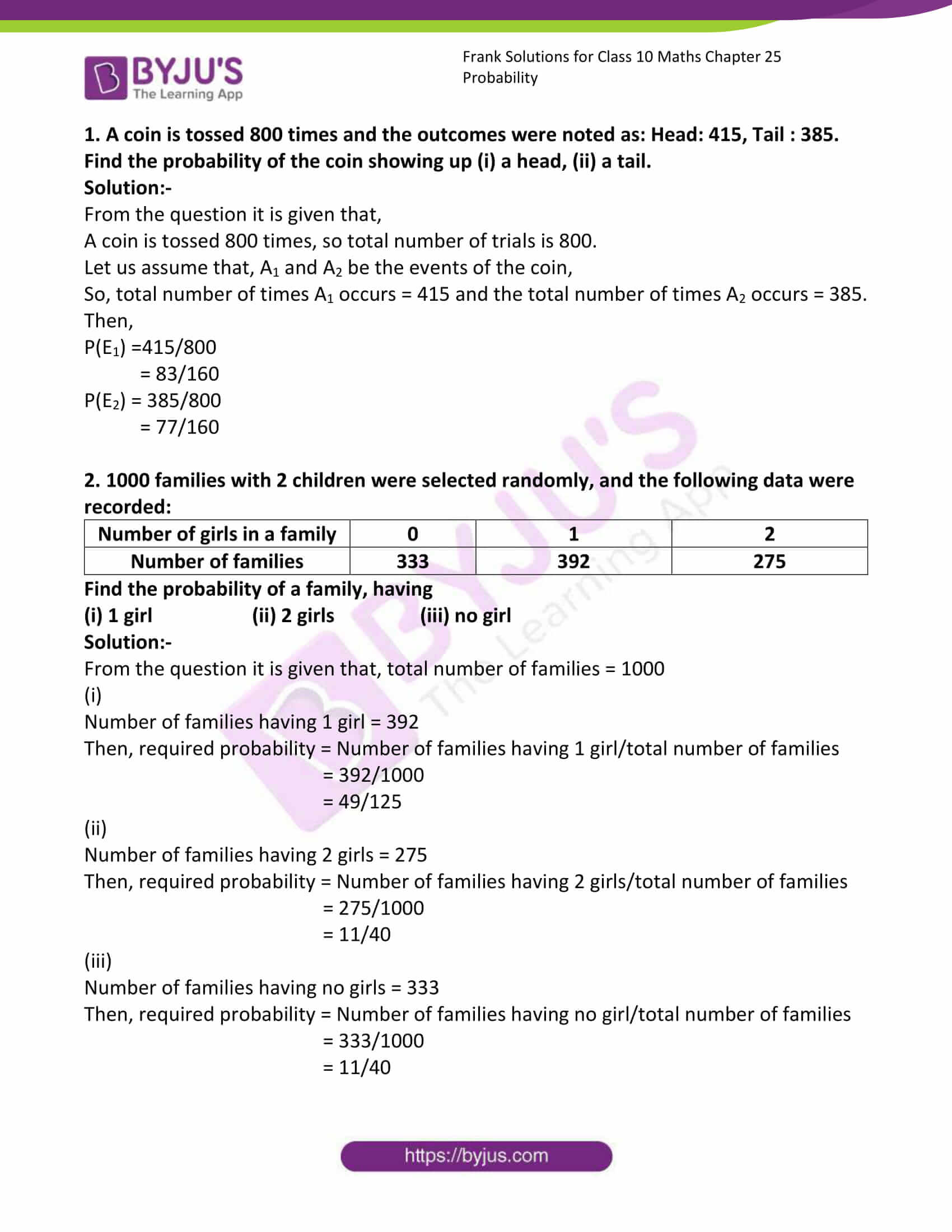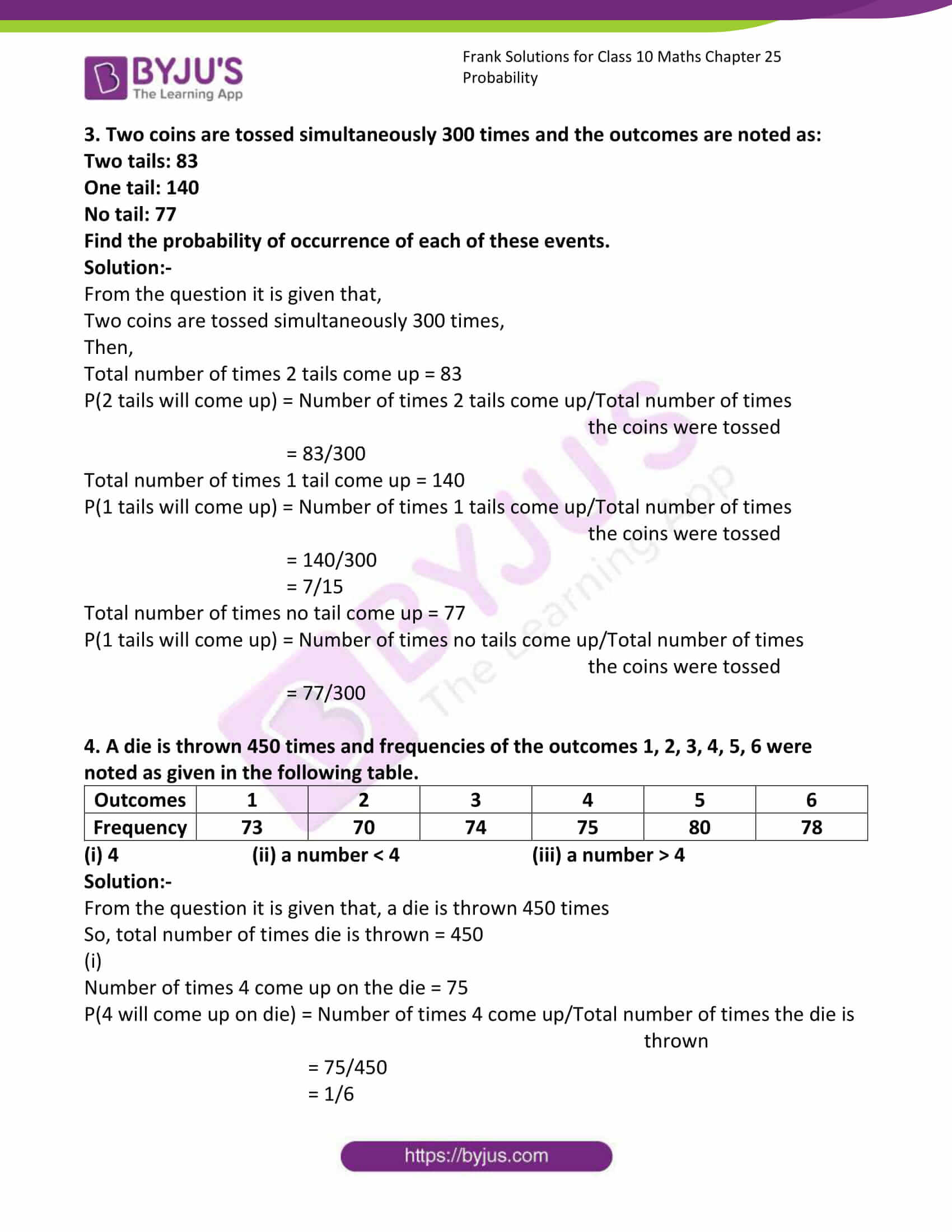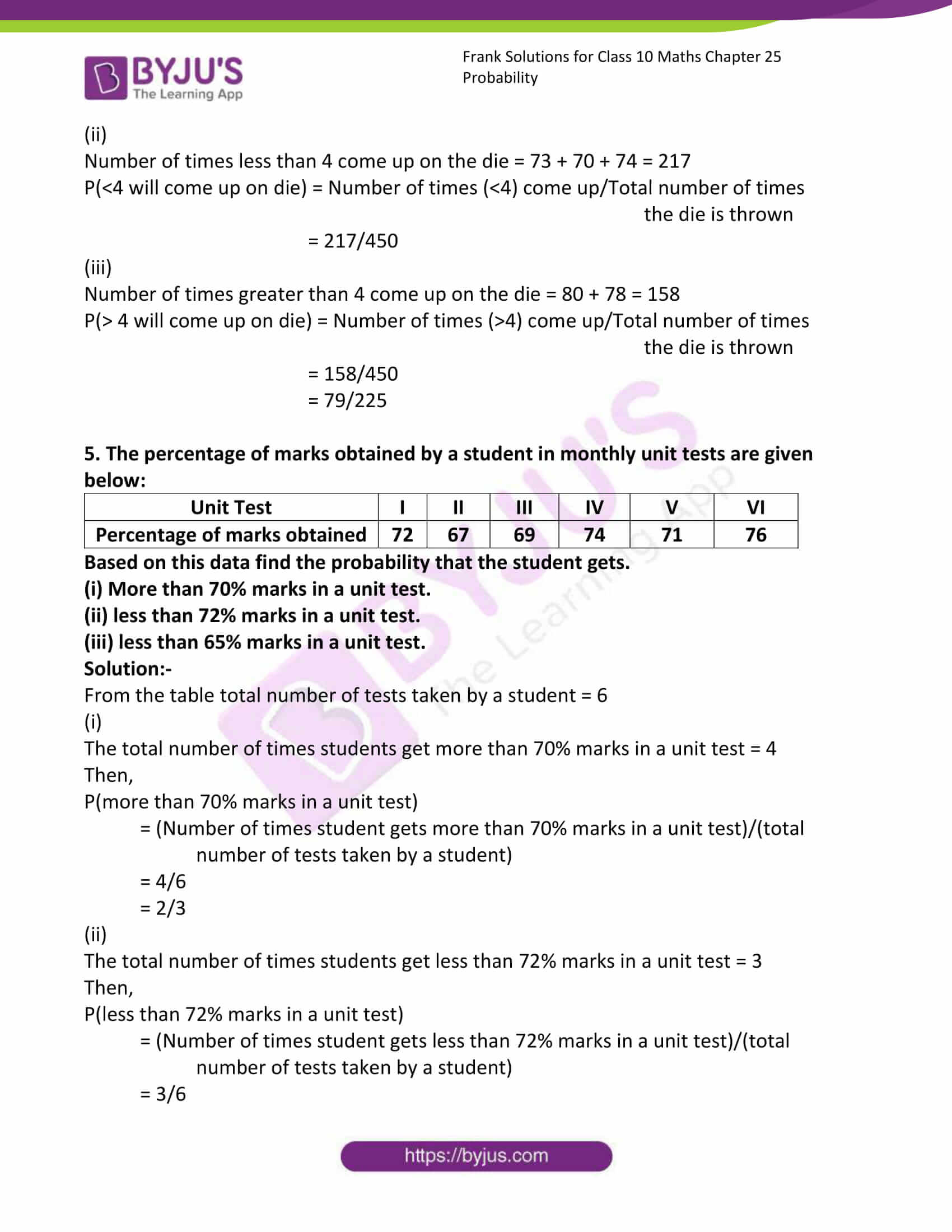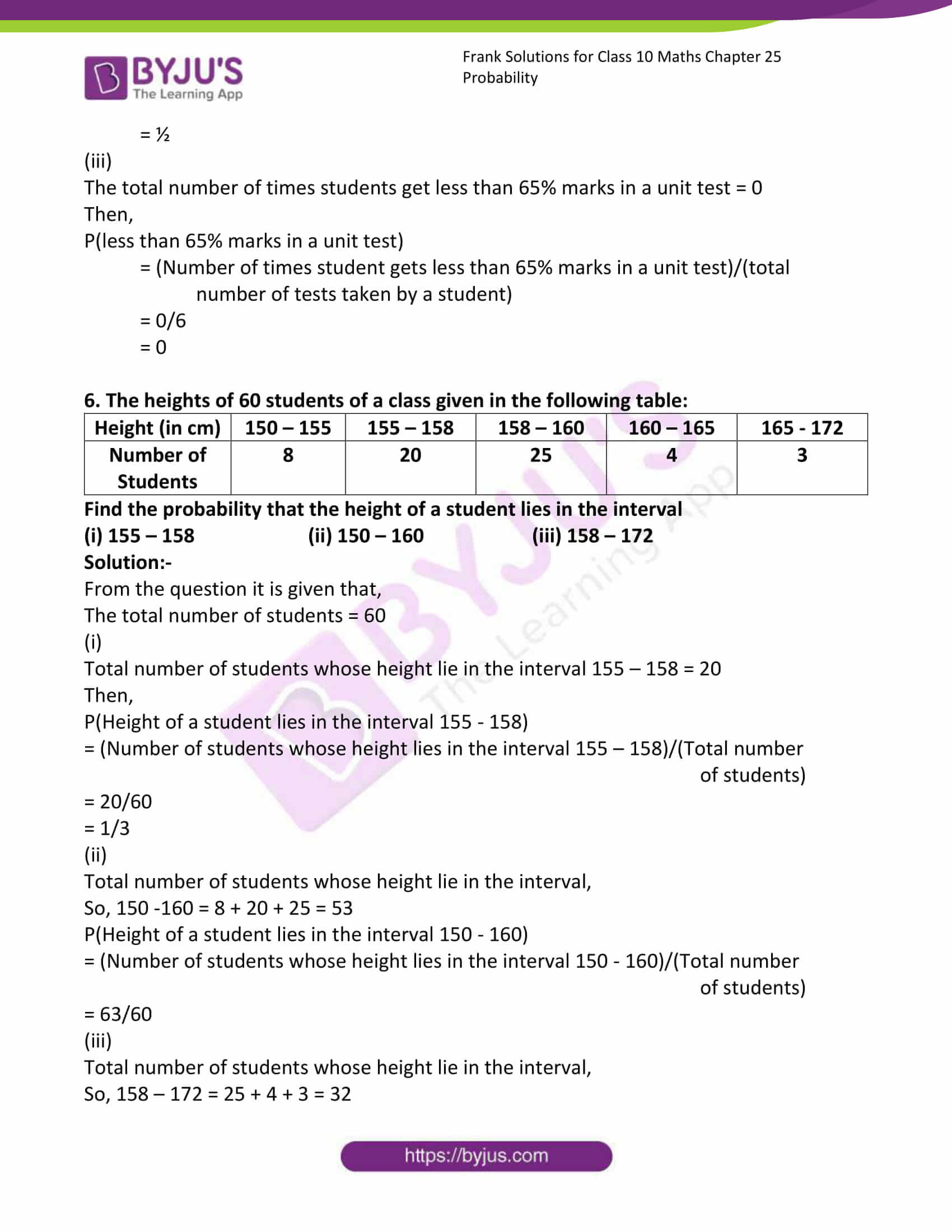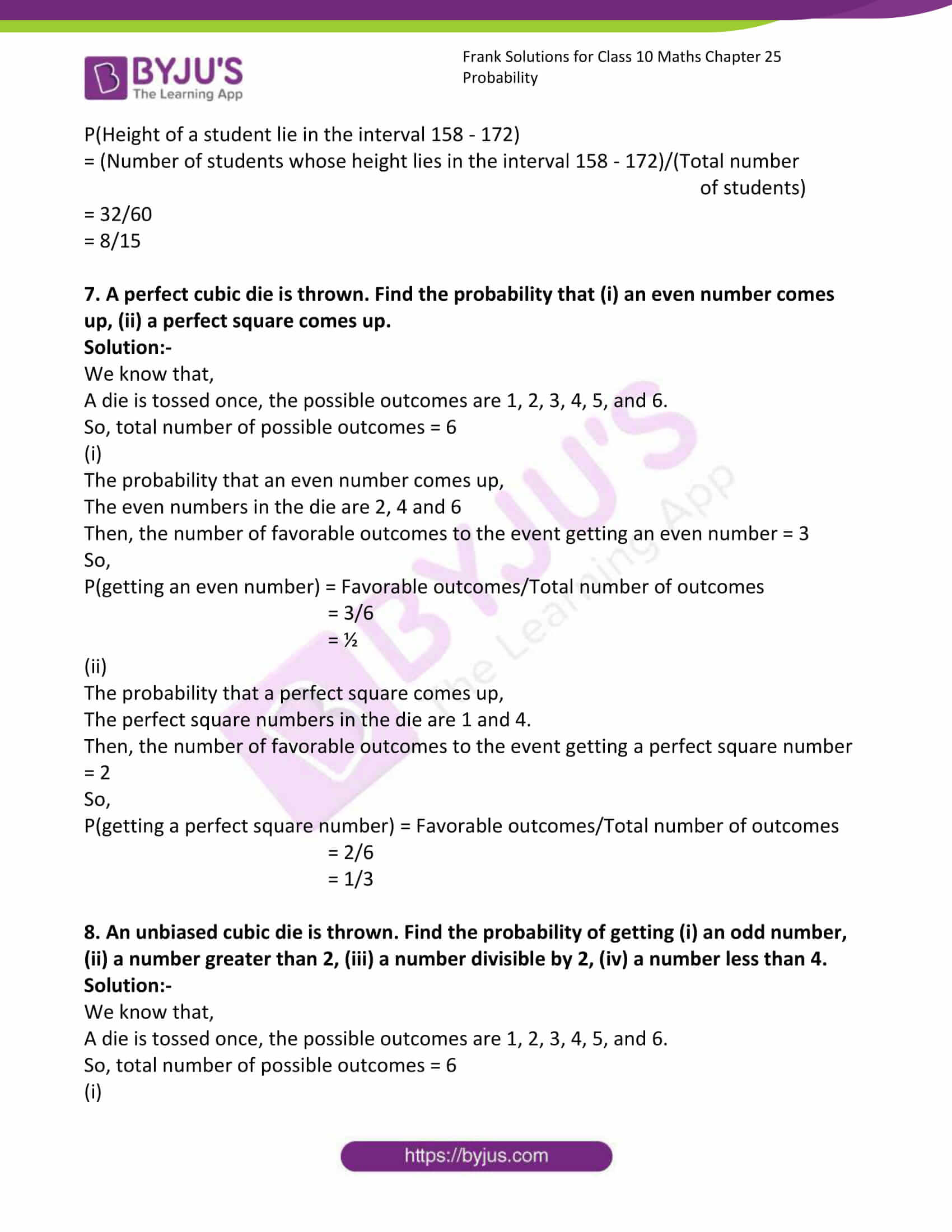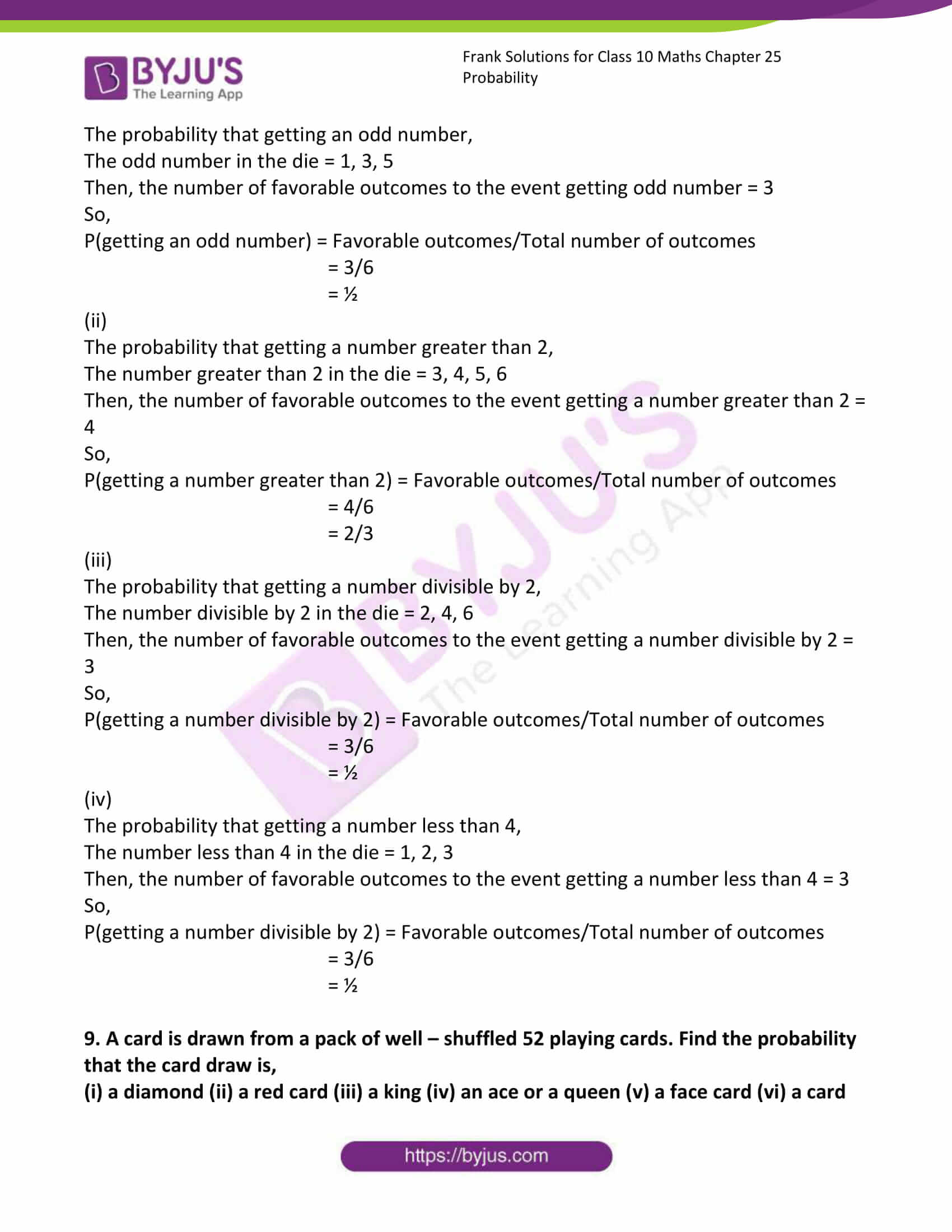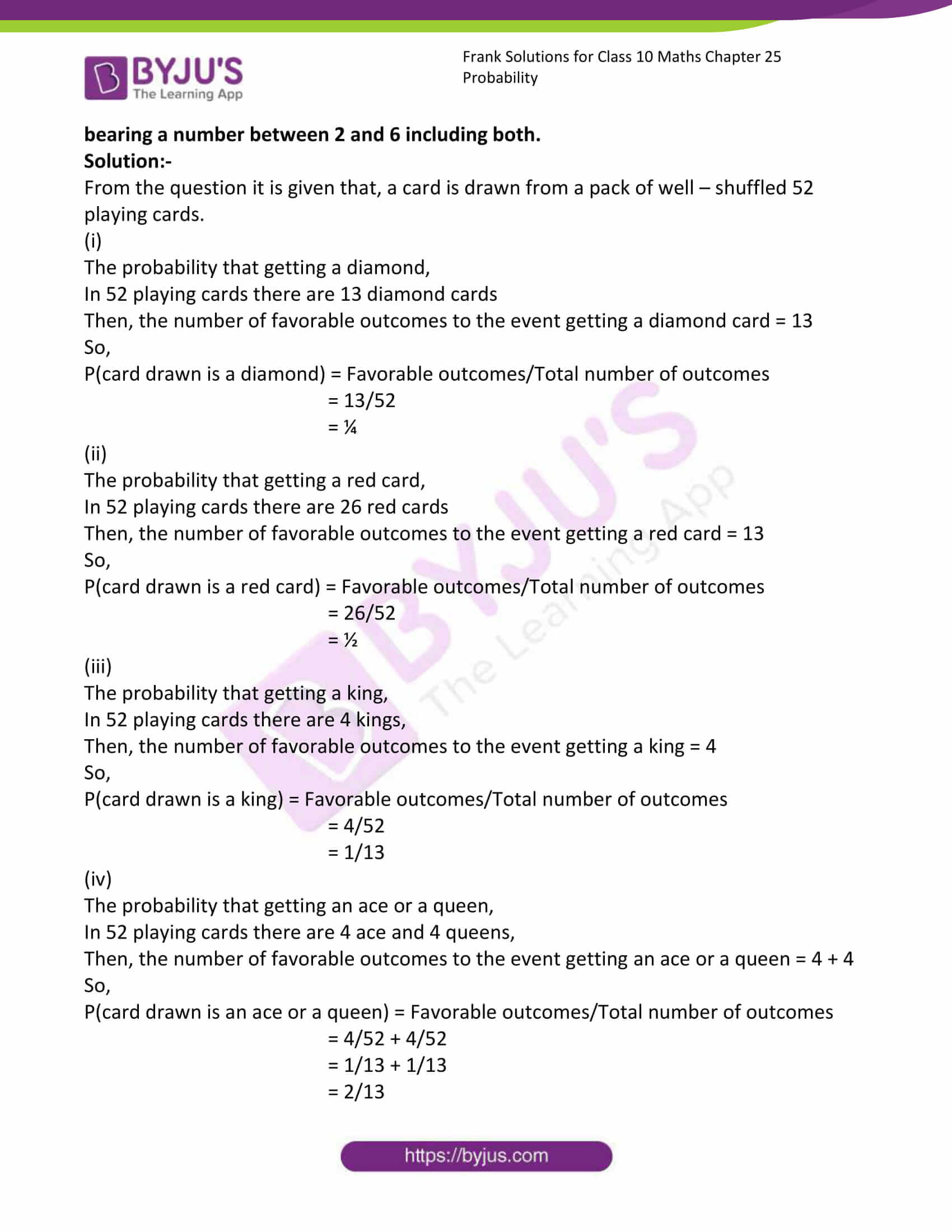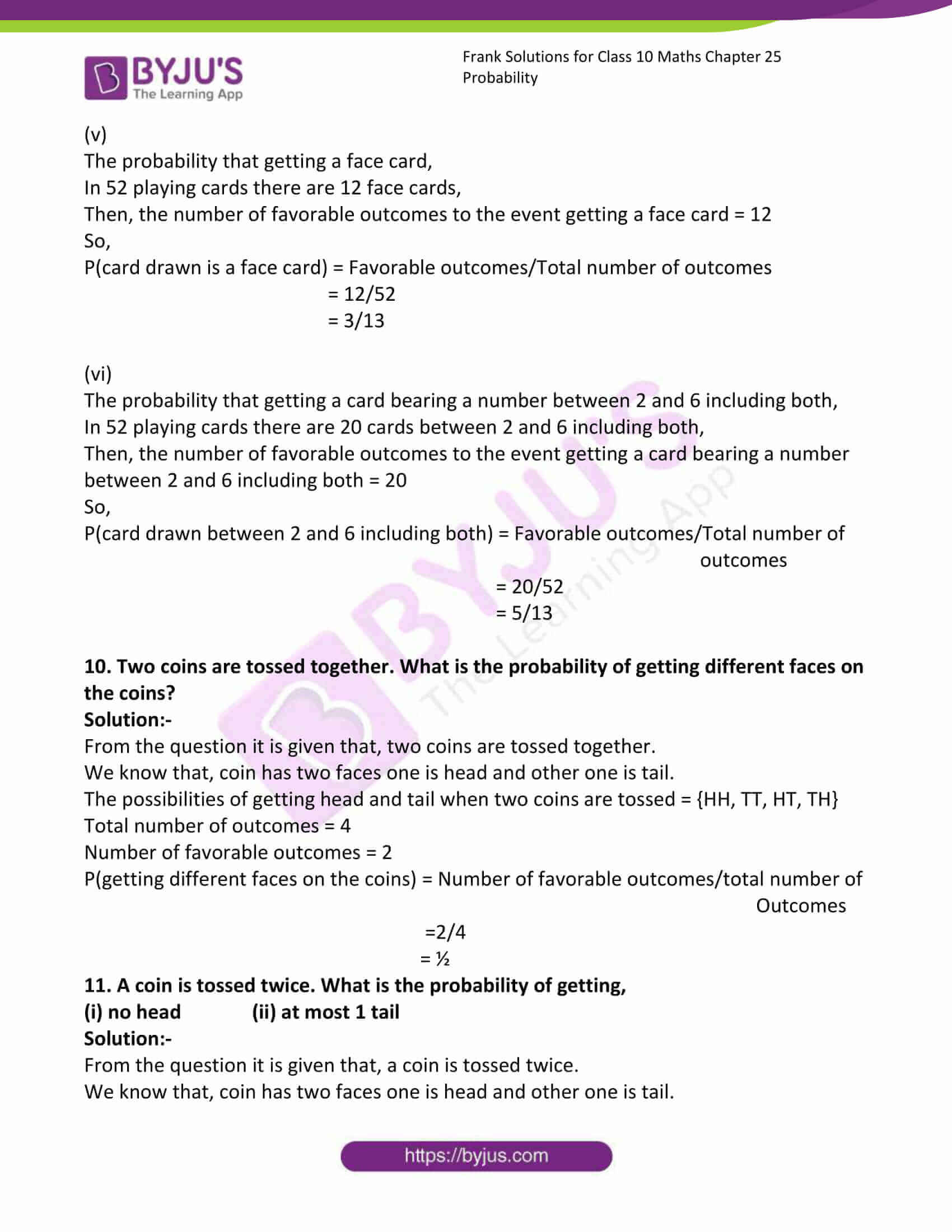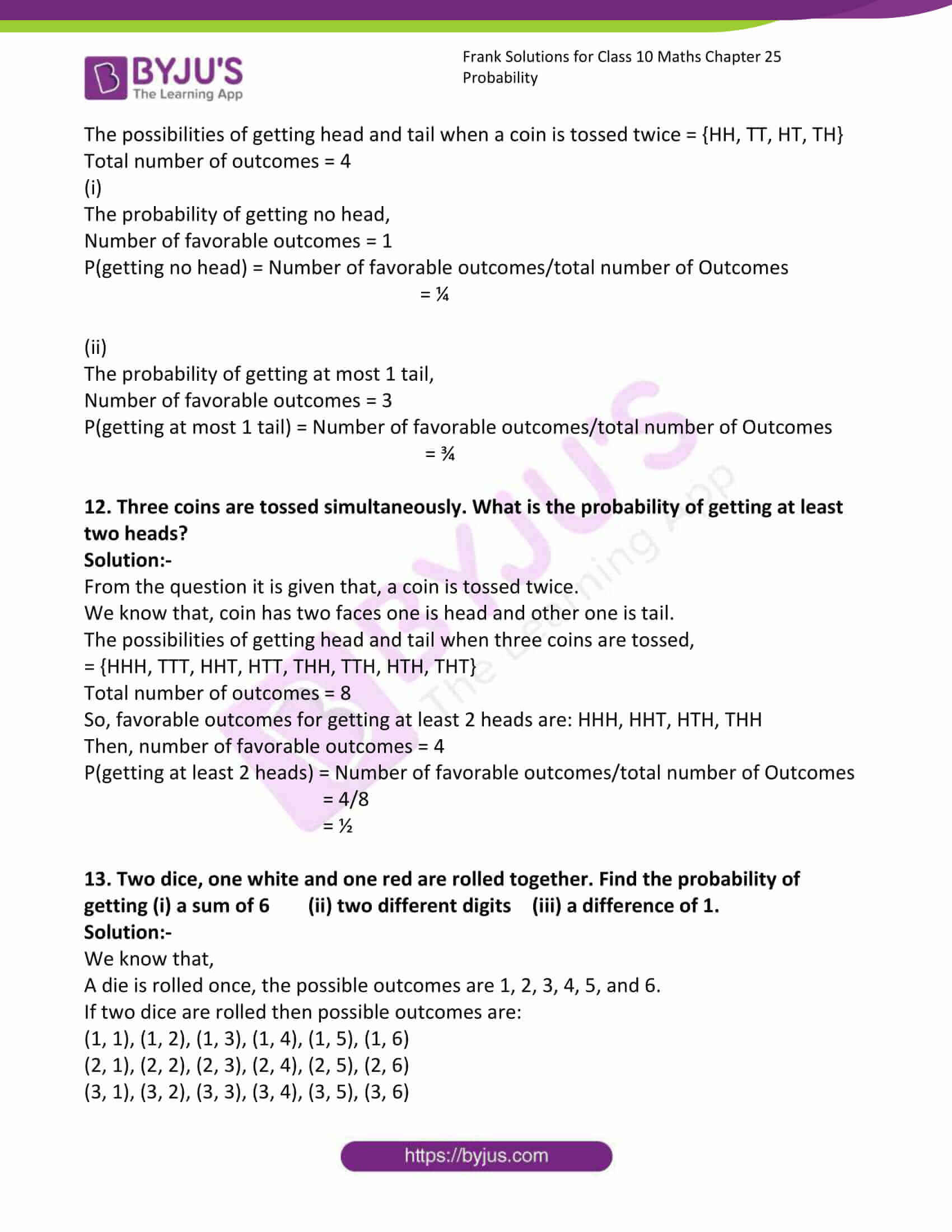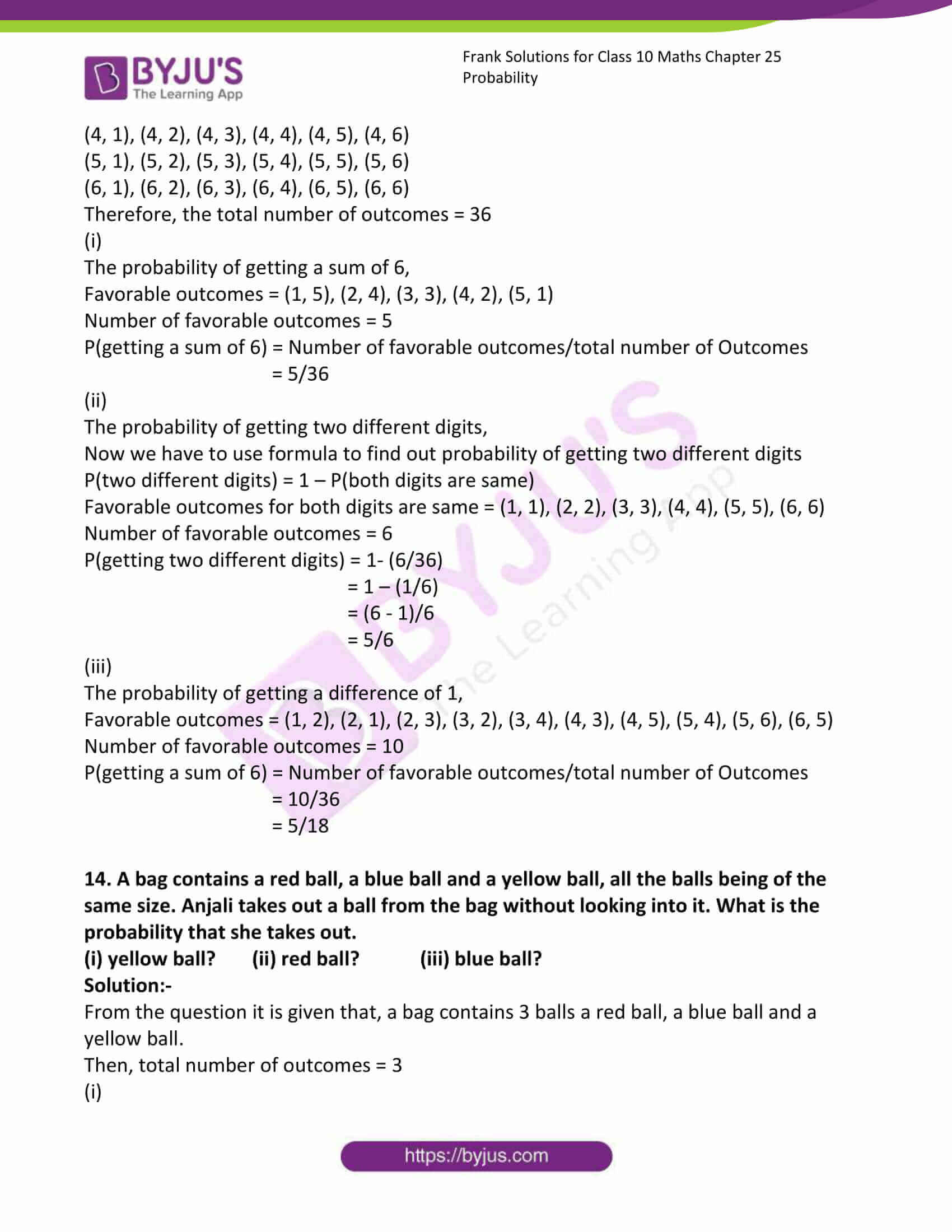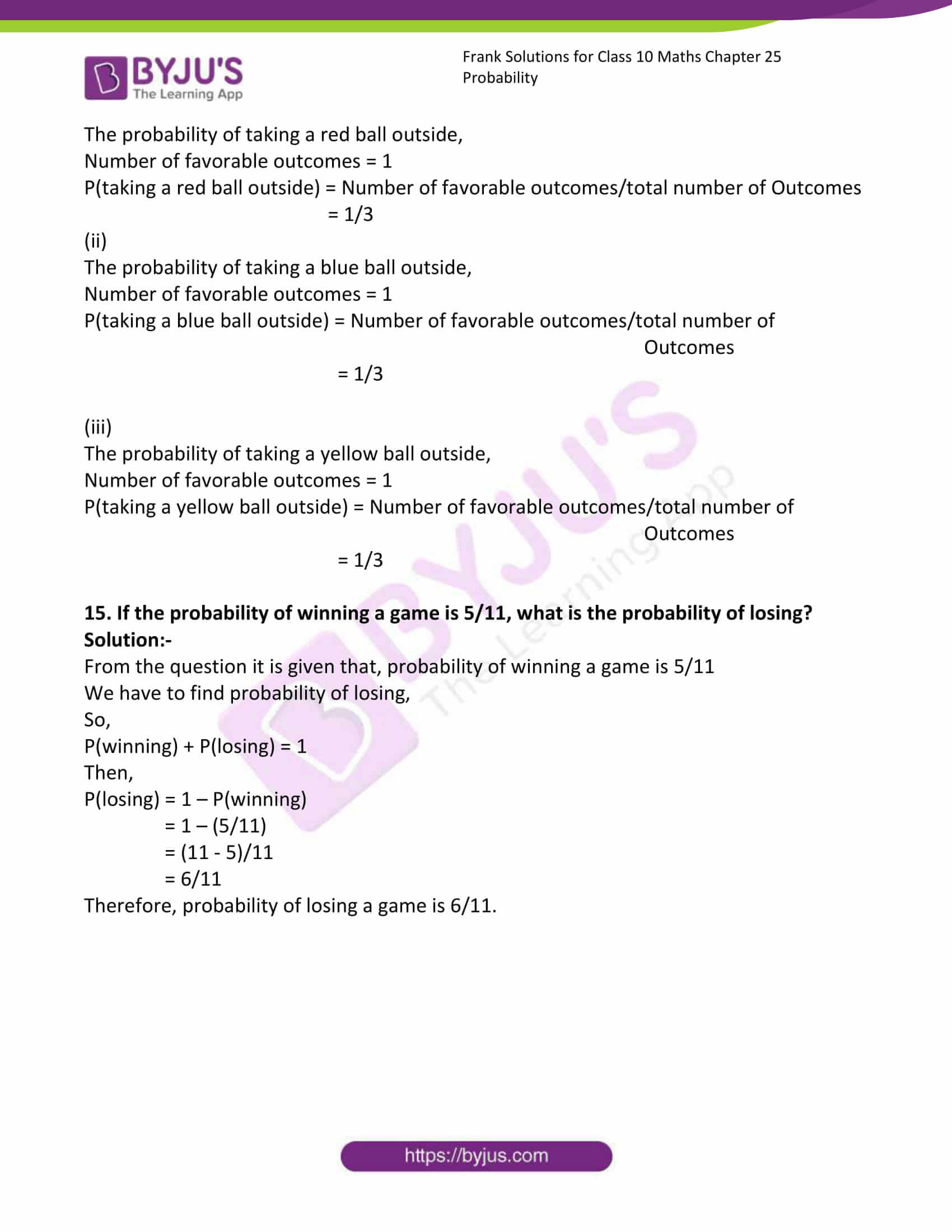### Access Answers to Frank Solutions for Class 10 Maths Chapter 25 Probability

1. A coin is tossed 800 times and the outcomes were noted as: Head: 415, Tail: 385. Find the probability of the coin showing up (i) a head, (ii) a tail.

Solution:-

From the question, it is given that,

A coin is tossed 800 times, so the total number of trials is 800.

Let us assume that, A1 and A2 be the events of the coin,

So, the total number of times A1 occurs = 415, and the total number of times A2 occurs = 385.

Then,

P(E1) =415/800

= 83/160

P(E2) = 385/800

= 77/160

2. 1000 families with 2 children were selected randomly, and the following data were recorded:

 Number of girls in a family 0 1 2 Number of families 333 392 275

Find the probability of a family having

(i) 1 girl (ii) 2 girls (iii) no girl

Solution:-

From the question, it is given that, the total number of families = 1000

(i)

Number of families having 1 girl = 392

Then, required probability = Number of families having 1 girl/total number of families

= 392/1000

= 49/125

(ii)

Number of families having 2 girls = 275

Then, required probability = Number of families having 2 girls/total number of families

= 275/1000

= 11/40

(iii)

Number of families having no girls = 333

Then, required probability = Number of families having no girl/total number of families

= 333/1000

= 11/40

3. Two coins are tossed simultaneously 300 times and the outcomes are noted as:

Two tails: 83

One tail: 140

No tail: 77

Find the probability of occurrence of each of these events.

Solution:-

From the question it is given that,

Two coins are tossed simultaneously 300 times,

Then,

Total number of times 2 tails come up = 83

P(2 tails will come up) = Number of times 2 tails come up/Total number of times

the coins were tossed

= 83/300

Total number of times 1 tail comes up = 140

P(1 tail will come up) = Number of times 1 tail comes up/Total number of times

the coins were tossed

= 140/300

= 7/15

Total number of times no tail comes up = 77

P(1 tail will come up) = Number of times no tails come up/Total number of times

the coins were tossed

= 77/300

4. A die is thrown 450 times and frequencies of the outcomes 1, 2, 3, 4, 5, 6 were noted as given in the following table.

 Outcomes 1 2 3 4 5 6 Frequency 73 70 74 75 80 78

(i) 4 (ii) a number < 4 (iii) a number > 4

Solution:-

From the question, it is given that, a die is thrown 450 times

So, the total number of times the die is thrown = 450

(i)

The number of times 4 comes up on the die = 75

P(4 will come up on die) = Number of times 4 come up/Total number of times the die is

thrown

= 75/450

= 1/6

(ii)

Number of times less than 4 come up on the die = 73 + 70 + 74 = 217

P(<4 will come up on die) = Number of times (<4) come up/Total number of times

the die is thrown

= 217/450

(iii)

Number of times greater than 4 come up on the die = 80 + 78 = 158

P(> 4 will come up on die) = Number of times (>4) come up/Total number of times

the die is thrown

= 158/450

= 79/225

5. The percentage of marks obtained by a student in monthly unit tests is given below:

 Unit Test I II III IV V VI Percentage of marks obtained 72 67 69 74 71 76

Based on this data, find the probability that the student gets.

(i) More than 70% marks in a unit test.

(ii) less than 72% marks in a unit test.

(iii) less than 65% marks in a unit test.

Solution:-

From the table total number of tests taken by a student = 6

(i)

The total number of times students get more than 70% marks in a unit test = 4

Then,

P(more than 70% marks in a unit test)

= (Number of times student gets more than 70% marks in a unit test)/(total

number of tests taken by a student)

= 4/6

= 2/3

(ii)

The total number of times students get less than 72% marks in a unit test = 3

Then,

P(less than 72% marks in a unit test)

= (Number of times student gets less than 72% marks in a unit test)/(total

number of tests taken by a student)

= 3/6

= ½

(iii)

The total number of times students get less than 65% marks in a unit test = 0

Then,

P(less than 65% marks in a unit test)

= (Number of times student gets less than 65% marks in a unit test)/(total

number of tests taken by a student)

= 0/6

= 0

6. The heights of 60 students in a class are given in the following table:

 Height (in cm) 150 – 155 155 – 158 158 – 160 160 – 165 165 – 172 Number of Students 8 20 25 4 3

Find the probability that the height of a student lies in the interval

(i) 155 – 158 (ii) 150 – 160 (iii) 158 – 172

Solution:-

From the question, it is given that,

The total number of students = 60

(i)

Total number of students whose height lies in the interval 155 – 158 = 20

Then,

P(Height of a student lies in the interval 155 – 158)

= (Number of students whose height lies in the interval 155 – 158)/(Total number

of students)

= 20/60

= 1/3

(ii)

Total number of students whose height lies in the interval,

So, 150 -160 = 8 + 20 + 25 = 53

P(Height of a student lies in the interval 150 – 160)

= (Number of students whose height lies in the interval 150 – 160)/(Total number

of students)

= 63/60

(iii)

Total number of students whose height lies in the interval,

So, 158 – 172 = 25 + 4 + 3 = 32

P(Height of a student lie in the interval 158 – 172)

= (Number of students whose height lies in the interval 158 – 172)/(Total number of students)

= 32/60

= 8/15

7. A perfect cubic die is thrown. Find the probability that (i) an even number comes up, (ii) a perfect square comes up.

Solution:-

We know that,

A die is tossed once, the possible outcomes are 1, 2, 3, 4, 5, and 6.

So, the total number of possible outcomes = 6

(i)

The probability that an even number comes up,

The even numbers in the die are 2, 4 and 6

Then, the number of favourable outcomes to the event getting an even number = 3

So,

P(getting an even number) = Favorable outcomes/Total number of outcomes

= 3/6

= ½

(ii)

The probability that a perfect square comes up,

The perfect square numbers in the die are 1 and 4.

Then, the number of favourable outcomes to the event getting a perfect square number = 2

So,

P(getting a perfect square number) = Favorable outcomes/Total number of outcomes

= 2/6

= 1/3

8. An unbiased cubic die is thrown. Find the probability of getting (i) an odd number, (ii) a number greater than 2, (iii) a number divisible by 2, (iv) a number less than 4.

Solution:-

We know that,

A die is tossed once, the possible outcomes are 1, 2, 3, 4, 5, and 6.

So, the total number of possible outcomes = 6

(i)

The probability that getting an odd number,

The odd number in the die = 1, 3, 5

Then, the number of favourable outcomes to the event getting an odd number = 3

So,

P(getting an odd number) = Favorable outcomes/Total number of outcomes

= 3/6

= ½

(ii)

The probability that getting a number greater than 2,

The number greater than 2 in the die = 3, 4, 5, 6

Then, the number of favourable outcomes to the event getting a number greater than 2 = 4

So,

P(getting a number greater than 2) = Favorable outcomes/Total number of outcomes

= 4/6

= 2/3

(iii)

The probability that getting a number divisible by 2,

The number divisible by 2 in the die = 2, 4, 6

Then, the number of favourable outcomes to the event getting a number divisible by 2 = 3

So,

P(getting a number divisible by 2) = Favorable outcomes/Total number of outcomes

= 3/6

= ½

(iv)

The probability that getting a number less than 4,

The number less than 4 in the die = 1, 2, 3

Then, the number of favourable outcomes to the event getting a number less than 4 = 3

So,

P(getting a number divisible by 2) = Favorable outcomes/Total number of outcomes

= 3/6

= ½

9. A card is drawn from a pack of well–shuffled 52 playing cards. Find the probability that the card draw is,

(i) a diamond (ii) a red card (iii) a king (iv) an ace or a queen (v) a face card (vi) a card bearing a number between 2 and 6, including both.

Solution:-

From the question, it is given that a card is drawn from a pack of well–shuffled 52 playing cards.

(i)

The probability that getting a diamond,

In 52 playing cards, there are 13 diamond cards

Then, the number of favourable outcomes to the event getting a diamond card = 13

So,

P(card drawn is a diamond) = Favorable outcomes/Total number of outcomes

= 13/52

= ¼

(ii)

The probability of getting a red card,

Of 52 playing cards, there are 26 red cards

Then, the number of favourable outcomes to the event getting a red card = 13

So,

P(card drawn is a red card) = Favorable outcomes/Total number of outcomes

= 26/52

= ½

(iii)

The probability that getting a king,

In 52 playing cards, there are 4 kings,

Then, the number of favourable outcomes to the event getting a king = 4

So,

P(card drawn is a king) = Favorable outcomes/Total number of outcomes

= 4/52

= 1/13

(iv)

The probability that getting an ace or a queen,

In 52 playing cards, there are 4 aces and 4 queens,

Then, the number of favourable outcomes to the event getting an ace or a queen = 4 + 4

So,

P(card drawn is an ace or a queen) = Favorable outcomes/Total number of outcomes

= 4/52 + 4/52

= 1/13 + 1/13

= 2/13

(v)

The probability that getting a face card,

In 52 playing cards, there are 12 face cards,

Then, the number of favourable outcomes to the event getting a face card = 12

So,

P(card drawn is a face card) = Favorable outcomes/Total number of outcomes

= 12/52

= 3/13

(vi)

The probability that getting a card bearing a number between 2 and 6, including both,

In 52 playing cards, there are 20 cards between 2 and 6, including both,

Then, the number of favourable outcomes to the event getting a card bearing a number between 2 and 6, including both = 20

So,

P(card drawn between 2 and 6 including both) = Favorable outcomes/Total number of

outcomes

= 20/52

= 5/13

10. Two coins are tossed together. What is the probability of getting different faces on the coins?

Solution:-

From the question, it is given that two coins are tossed together.

We know that the coin has two faces; one is the head, and the other one is the tail.

The possibilities of getting head and tail when two coins are tossed = {HH, TT, HT, TH}

Total number of outcomes = 4

Number of favourable outcomes = 2

P(getting different faces on the coins) = Number of favourable outcomes/total number of

Outcomes

=2/4

= ½

11. A coin is tossed twice. What is the probability of getting,

(i) no head (ii) at most 1 tail

Solution:-

From the question, it is given that a coin is tossed twice.

We know that the coin has two faces; one is the head, and the other one is the tail.

The possibilities of getting head and tail when a coin is tossed twice = {HH, TT, HT, TH}

Total number of outcomes = 4

(i)

The probability of getting no head,

Number of favourable outcomes = 1

P(getting no head) = Number of favourable outcomes/total number of Outcomes

= ¼

(ii)

The probability of getting at most 1 tail,

Number of favourable outcomes = 3

P(getting at most 1 tail) = Number of favourable outcomes/total number of Outcomes

= ¾

12. Three coins are tossed simultaneously. What is the probability of getting at least two heads?

Solution:-

From the question, it is given that a coin is tossed twice.

We know that the coin has two faces; one is the head, and the other one is the tail.

The possibilities of getting head and tail when three coins are tossed,

= {HHH, TTT, HHT, HTT, THH, TTH, HTH, THT}

Total number of outcomes = 8

So, favourable outcomes for getting at least 2 heads are: HHH, HHT, HTH, THH

Then, the number of favourable outcomes = 4

P(getting at least 2 heads) = Number of favourable outcomes/total number of Outcomes

= 4/8

= ½

13. Two dice, one white and one red, are rolled together. Find the probability of getting (i) a sum of 6 (ii) two different digits (iii) a difference of 1.

Solution:-

We know that,

A die is rolled once, the possible outcomes are 1, 2, 3, 4, 5, and 6.

If two dice are rolled, then the possible outcomes are:

(1, 1), (1, 2), (1, 3), (1, 4), (1, 5), (1, 6)

(2, 1), (2, 2), (2, 3), (2, 4), (2, 5), (2, 6)

(3, 1), (3, 2), (3, 3), (3, 4), (3, 5), (3, 6)

(4, 1), (4, 2), (4, 3), (4, 4), (4, 5), (4, 6)

(5, 1), (5, 2), (5, 3), (5, 4), (5, 5), (5, 6)

(6, 1), (6, 2), (6, 3), (6, 4), (6, 5), (6, 6)

Therefore, the total number of outcomes = 36

(i)

The probability of getting a sum of 6,

Favourable outcomes = (1, 5), (2, 4), (3, 3), (4, 2), (5, 1)

Number of favourable outcomes = 5

P(getting a sum of 6) = Number of favourable outcomes/total number of Outcomes

= 5/36

(ii)

The probability of getting two different digits,

Now we have to use the formula to find out the probability of getting two different digits

P(two different digits) = 1 – P(both digits are same)

Favourable outcomes for both digits are the same = (1, 1), (2, 2), (3, 3), (4, 4), (5, 5), (6, 6)

Number of favourable outcomes = 6

P(getting two different digits) = 1- (6/36)

= 1 – (1/6)

= (6 – 1)/6

= 5/6

(iii)

The probability of getting a difference of 1,

Favourable outcomes = (1, 2), (2, 1), (2, 3), (3, 2), (3, 4), (4, 3), (4, 5), (5, 4), (5, 6), (6, 5)

Number of favourable outcomes = 10

P(getting a sum of 6) = Number of favourable outcomes/total number of Outcomes

= 10/36

= 5/18

14. A bag contains a red ball, a blue ball and a yellow ball, all the balls being of the same size. Anjali takes out a ball from the bag without looking into it. What is the probability that she takes it out?

(i) yellow ball? (ii) red ball? (iii) blue ball?

Solution:-

From the question, it is given that a bag contains 3 balls a red ball, a blue ball and a yellow ball.

Then, the total number of outcomes = 3

(i)

The probability of taking a red ball outside,

Number of favourable outcomes = 1

P(taking a red ball outside) = Number of favourable outcomes/total number of Outcomes

= 1/3

(ii)

The probability of taking a blue ball outside,

Number of favourable outcomes = 1

P(taking a blue ball outside) = Number of favourable outcomes/total number of

Outcomes

= 1/3

(iii)

The probability of taking a yellow ball outside,

Number of favourable outcomes = 1

P(taking a yellow ball outside) = Number of favourable outcomes/total number of

Outcomes

= 1/3

15. If the probability of winning a game is 5/11, what is the probability of losing?

Solution:-

From the question, it is given that the probability of winning a game is 5/11

We have to find the probability of losing,

So,

P(winning) + P(losing) = 1

Then,

P(losing) = 1 – P(winning)

= 1 – (5/11)

= (11 – 5)/11

= 6/11

Therefore, the probability of losing a game is 6/11.